# PSAT Math : How to find if acute / obtuse triangles are congruent

## Example Questions

### Example Question #1 : Acute / Obtuse Triangles

You are given triangles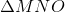and, with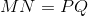and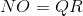. Which of these statements, along with what you are given, is not enough to prove that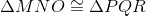?

I)and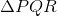have the same perimeter

II)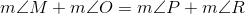III)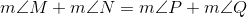Statement I only

None of these statements is enough to prove congruence.

Statement III only

Any of the three statements is enough to prove congruence.

Statement II only

Statement III only

Explanation:

Ifandhave the same perimeter,, and, it follows that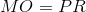. The three triangles have the same sidelengths, setting the conditions for the Side-Side-Side Congruence Postulate.

If, then, since the sum of the degree measures of both triangles is the same (180 degrees), it follows that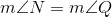. Since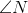and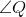are congruent included angles of congruent sides, this sets the conditions for the SAS Congruence Postulate.

In both of the above cases, it follows that.

However, similarly to the previous situation, if, then it follows that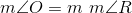, meaning that we have congruent sides and congruent nonincluded angles. However, this is not sufficient to prove congruence.

"Statement III" is the correct response.